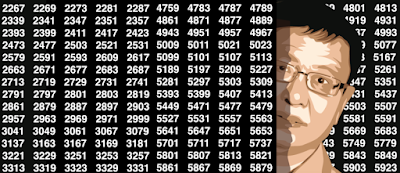## Sunday, November 19, 2017

### High Precision Computing: Benchmark, Examples, and Tutorial

In some applications, using the standard precision in your programming language of choice, may not be enough, and can lead to disastrous errors. In some cases, you work with a library that is supposed to provide very high precision, when in fact the library in question does not work as advertised. In some cases, lack of precision results in obvious problems that are easy to spot, and in some cases, everything seems to be working fine and you are not aware that your simulations are completely wrong after as little as 30 iterations. We explore this case in this article, using a simple example that can be used to test the precision of your tool and of your results.
Such problems arise frequently with algorithms that do not converge to a fixed solution, but instead generate numbers that oscillate continuously in some interval, converging in distribution rather than in value, unlike traditional algorithms that aim to optimize some function. The examples abound in chaotic theory, and the simplest case is the recursion X(k + 1) = 4 X(k) (1- X(k)), starting with a seed s = X(0) in [0, 1]. We will use this example - known as the logistic map - to benchmark various computing systems.
Examples of algorithms that can be severely impacted by aggregated loss of precision, besides ill-conditioned problems, include:
• Markov Chain Monte Carlo (MCMC) simulations, a modern statistical method of estimation for complex problems and nested or hierarchical models, including Bayesian networks.
• Reflective stochastic processes, see here. This includes some some types or Brownian or Wiener processes.
• Chaotic processes, see here (especially section 2.) These include fractals.
• Continuous random number generators, see here.
The conclusions based on the faulty sequences generated are not necessarily invalid, as long as the focus is on the distribution being studied, rather than on the exact values from specific sequences.

## Tuesday, November 7, 2017

### Fascinating Chaotic Sequences with Cool Applications

Here we describe well-known chaotic sequences, including new generalizations, with application to random number generation, highly non-linear auto-regressive models for times series, simulation, random permutations, and the use of big numbers (libraries available in programming languages to work with numbers with hundreds of decimals) as standard computer precision almost always produces completely erroneous results after a few iterations  -- a fact rarely if ever mentioned in the scientific literature, but illustrated here, together with a solution. It is possible that all scientists who published on chaotic processes, used faulty numbers because of this issue.
This article is accessible to non-experts, even though we solve a special stochastic equation for the first time, providing an unexpected exact solution, for a new chaotic process that generalizes the logistic map. We also describe a general framework for continuous random number generators, and investigate the interesting auto-correlation structure associated with some of these sequences. References are provided, as well as fast source code to process big numbers accurately, and even an elegant mathematical proof in the last section.This article is also a useful read for participants in our upcoming competition (to be announced soon) as it addresses a similar stochastic integral equation problem, also with exact solution, in the related context of self-correcting random walks - another kind of memory-less process.
The approach used here starts with traditional data science and simulations for exploratory analysis, with empirical results confirmed later by mathematical arguments in the last section.

### Machine Learning Perspective on the Twin Prime Conjecture

This article focuses on the machine learning aspects of the problem, and the use of pattern recognition techniques leading to interesting,...